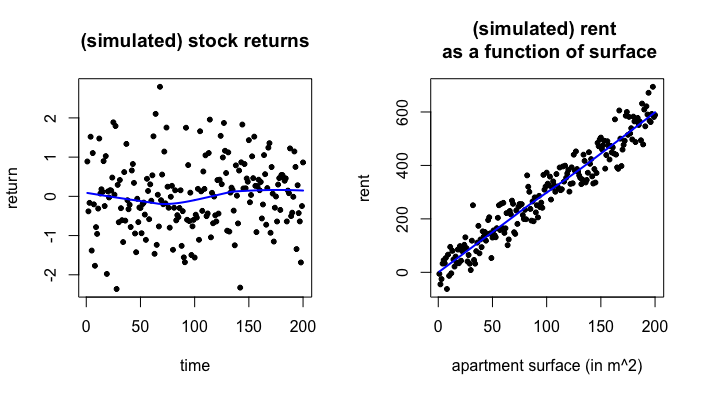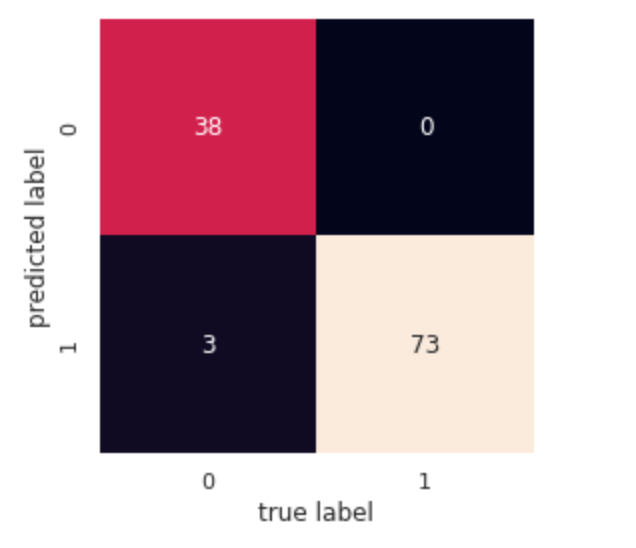Two variants of Adaboost (Adaptive boosting) algorithms are now included in the development version of nnetsauce, available on Github. My nnetsauce implementation of Adaboost has some specificities, as it will be shown in the sequel of this post. It is also worth noting that the current implementation is 100% Python (neither underlying C, nor C++).

The package can be imported from Github, by doing:

pip install git+https://github.com/Techtonique/nnetsauce.git


I’ll show you how to use these Adaboost classifiers on two popular datasets.

First, a few words about statistical/machine learning (ML hereafter). ML is about pattern recognition. A phenomenon that has a trend or a seasonality, such as the evolution of the weather, can be studied by ML. Other use cases include identifying fraudulent transactions (unless, of course, the smarts increase at a dramatically fast pace), determining if a tumor is benign or malignant, natural language processing, etc. On the other hand ML cannot say which of heads or tail will appear next when you flip a fair coin. By using statistical inference, you can derive quantities such as the probability of the number of trials until head or tails appear, but that’s it.

Another illustration is presented below. All that I can say about my simulated stock returns (on the left), is that their average is 0, and their standard deviation is 1. Trying to predict the next return will (extremely) likely give me: 0. On the right, I can see a trend in my simulated rents. So, I can predict more or less accurately the rent of an appartment; assuming that an increase of 1 squared meter in and appartment’s surface produces an increase of 3€ in rents.Adaboost is an ML algorithm, i.e it achieves pattern recognition. More specifically, it’s an ensemble learning algorithm called boosting. For more details about boosting in general, the interested reader can consult this paper. And for Adaboost in particular, that one. The aim of ensemble learning is to combine multiple individual ML models into one. Ensembling thus aims at obtaining a model, that has an improved recognition error over the individual models’ recognition error. And most of the times, it works.

We start by importing the packages necessary for the job, along with nnetsauce (namely numpy and sklearn, nothing weird!):

import nnetsauce as ns
import numpy as np
from sklearn.linear_model import LogisticRegression
from sklearn.model_selection import train_test_split
from sklearn import metrics


Our first example is based on wisconsin breast cancer dataset from UCI (University of California at Irvine) repository, and available in sklearn. More details about the content of these datasets can be found here and here. wisconsin breast cancer dataset is splitted into a training set (for training the model to pattern recognition) and test set (for model validation):

# Import dataset from sklearn
X = breast_cancer.data
y = breast_cancer.target

# training test and test set
X_train, X_test, y_train, y_test = train_test_split(X, y, test_size=0.2,
random_state=123)


The first version of Adaboost that we apply is SAMME.R, also known as Real Adaboost. The acronym SAMME stands for Stagewise Additive Modeling using a Multi-class Exponential loss function, and nnetsauce’s implementation of SAMME has some specificities:

-The base learners (individual models in the ensemble) are quasi-randomized (deterministic) networks.

-At each boosting iteration, a fraction of dataset’s observations can be randomly chosen, in order to increase diversity within the ensemble.

-For SAMME (not for SAMME.R, yet), an experimental feature allows to apply an elastic net-like constraint to individual observations weights. That is: the norm of these individual weights can be bounded during the learning procedure. I am curious to hear how well (or not) it works for you.

# SAMME.R

# base learner
clf = LogisticRegression(solver='liblinear', multi_class = 'ovr',
random_state=123)

n_hidden_features=11,
n_estimators=250, learning_rate=0.01126343,
col_sample=0.72684326, row_sample=0.86429443,
dropout=0.63078613, n_clusters=2,
type_clust="gmm",
verbose=1, seed = 123,
method="SAMME.R")



The base learner, clf, is a logistic regression model but it could be anything including decision trees. fit_obj is a nnetsauce object that augments clf with a hidden layer of transformed predictors, and typically makes clf’s predictions nonlinear. n_hidden_features is the number of nodes in the hidden layer, and dropout randomly drops some of these nodes at each boosting iteration (which reduces overtraining). col_sample and row_sample specify the fraction of columns and rows chosen for fitting the base learner at each iteration. With n_clusters, the data can be clustered into homogeneous groups before model training.

nnetsauce’s Adaboost can now be fitted; 250 iterations are used:

# Fitting the model to training set
fit_obj.fit(X_train, y_train)

# Obtain model's accuracy on test set
print(fit_obj.score(X_test, y_test))


With the following graph, we can visualize how well our data have been classified by nnetsauce’s Adaboost.

import matplotlib.pyplot as plt
import seaborn as sns; sns.set()
from sklearn.metrics import confusion_matrix
preds = fit_obj.predict(X_test)
mat = confusion_matrix(y_test, preds)
sns.heatmap(mat.T, square=True, annot=True, fmt='d', cbar=False)
plt.xlabel('true label')
plt.ylabel('predicted label');1 denotes a malignant tumor, and 0, its absence. For the 3 (out of 114) patients remaining missclassified, it could be interesting to change the model sample_weights, and give them more weight in the learning procedure. Then, we could see how well the result evolves with this change; depending on which classifier’s decision we consider being the worst (or best). But note that:

1.The model will never be perfect (plus, the labels are based on human-eyed labelling ;) ). Still: though he said “all models are wrong”, he didn’t mean “are false”. He meant wrong in the sense that these are simply (even sometimes, great) representations of a reality. “False” would be: wrong to an extent that can’t be tolerated. And indeed in that regard, some models are false, for certain purposes. If I fit a model to this dataset and get an accuracy of 30%, no matter how sophisticated or expensive it is, the model is just plainly unacceptable - for that purpose.

2.Patients are not labelled. Label is just a generic term in classification, for all types of classification models and data. Here, those are 0 and 1.

Our second example is based on wine dataset from UCI repository. This dataset contains information about wines’ quality, depending on their characteristics. With ML applied to this dataset, we can deduce the quality of a wine, previously unseen, by using its characteristics. SAMME is now used instead of SAMME.R. This second algorithm seems to require more iterations to converge than SAMME.R (but you, tell me from your experience!):

# load dataset
Z = wine.data
t = wine.target
np.random.seed(123)
Z_train, Z_test, y_train, y_test = train_test_split(Z, t, test_size=0.2)

# SAMME
clf = LogisticRegression(solver='liblinear', multi_class = 'ovr',
random_state=123)
n_hidden_features=np.int(8.21154785e+01),
n_estimators=1000, learning_rate=2.96252441e-02,
col_sample=4.22766113e-01, row_sample=7.87268066e-01,
dropout=1.56909180e-01, n_clusters=3,
type_clust="gmm",
verbose=1, seed = 123,
method="SAMME")

# Fitting the model to training set
fit_obj.fit(Z_train, y_train)


After fitting the model, we can obtain some statistics about its quality (accuracy, precision, recall, f1-score; every nnetsauce model is 100% sklearn-compatible) in classifying unseen wines:

# model predictions on unseen wines
preds = fit_obj.predict(Z_test)

# descriptive statistics of model performance
print(metrics.classification_report(preds, y_test))


A Jupyter notebook for this post can be found here. More examples of use of nnetsauce’s Adaboost here.

Note: I am currently looking for a side hustle. You can hire me on Malt or send me an email: thierry dot moudiki at pm dot me. I can do descriptive statistics, data preparation, feature engineering, model calibration, training and validation, and model outputs’ interpretation. I am fluent in Python, R, SQL, Microsoft Excel, Visual Basic (among others) and French. My résumé? Here!